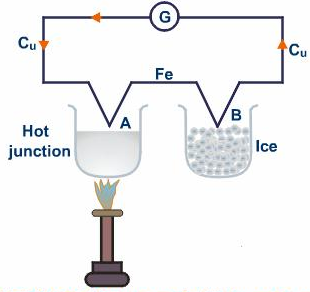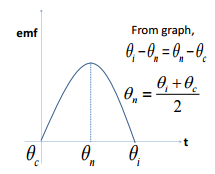# Variation of Thermo-emf with Temperature

The thermo emf generated by the thermocouple varies with temperature.  So, the temperature is maintained in such a way that there will be generation of maximum emf. The temperature at which there is a generation of maximum emf is called neutral temperature. For this, the temperature of one of the junction is kept constant. On increasing further temperature, the thermoemf decreases and again becomes zero. After this, the emf becomes negative. This is called temperature of inversion.Apparatus for variation of thermo-emf with temperature

Let us consider an iron-copper thermocouple is taken and fitted with a galvanometer as shown in figure. Here, one junction is kept in oil bath and another is immersed in ice. The temperature of ice is kept constant. When the temperature of both junction is same, the galvanometer shows null deflection as there is no generation of thermoemf.

Now, the temperature of hot junction is made increased by heating the oil bath provided with the burner.  The temperature of hot junction is increased by keeping the temperature of cold junction constant. At certain temperature, the galvanometer shows the maximum deflection.  This temperature is called the neutral temperature. On increasing the temperature further, the deflection of galvanometer goes on decreasing.

The variation of thermo-emf with temperature is given by:

$$E = \alpha \theta + \frac{1}{2} \beta \theta ^2$$

Where θ is the temperature, α and β are constants which depends upon the material of the conductor and temperature difference of two junctions.

Let To be the temperature of the cold junction, Tn be the neutral temperature and Ti be the temperature of inversion. Now, since the temperature of inversion is greater than the neutral temperature, we can write:

∴ Ti – Tn = Tn – To

or, Tn = (Ti +To)/2

This is the required relation.Graph of thermo-emf vs temperature

When a curve is plotted for temperature vs emf, we obtain a curve line as shown in figure. This shows that the thermoemf increases with the increase in temperature, becomes maximum at certain value and decreases again.

Do you like this article ? If yes then like otherwise dislike : 1